# Electronics and Communication Engineering - Exam Questions Papers

11.

Which of the following equations satisfy Maxwell's equations?

 A. Ex = Ey = 0, Ez = cos (y - ct)Bx = cos(y - ct), By = Bz = 0 B. Ex = Ey = 0, Ez = cos (y- ct)By = cos(y - ct), Bx = Bz = 0 C. Fy = cos (y - ct), Ex = Ez = 0Bx = cos(y - ct), By = Bz = 0 D. None of these

Explanation: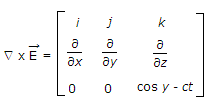=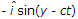and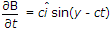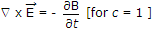.

12.

Conversion rate, for a successive approximation ADC which uses 2 MHz clock and 5 bit binary ladder containing 8 VV reference voltage is __________ .

 A. 64516.12 conversions/sec B. 400000 conversions/sec C. 400000 bps D. 2.5 bps

Explanation:

Conversion rate =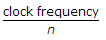=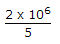= 400000 conversions/sec.

13.

V(t) across R2 for t > 0 is given by,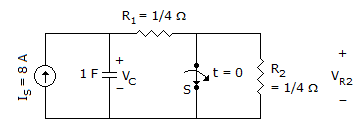A. 2 - 2e-2t B. 2 - e-2t C. 2 - e-t D. 1 - e-t

Explanation:

Time constant t = RC

Req for t > 0 is; R1 = R2 =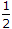C = 1F

t = 1/2

V(t) = VF - (VF - Vi)e-t/z

VF = VR2(t = ∞) = 2 V

Vi = VR2 (t = 0+) = 1 V

V(t) = 2 - (2 - 1)e-t/1/2

V(t) = 2 - e-2t.

14.

The rms value of the periodic waveform e(t) shown in fig. is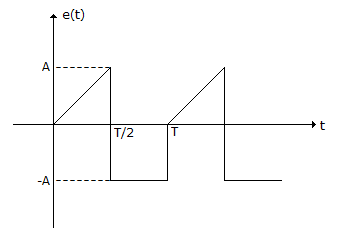A.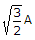B.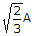C.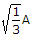D. 3A

Explanation: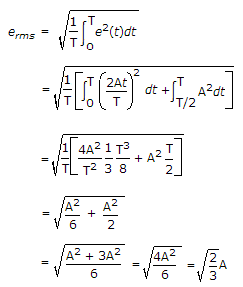15.

A parallel plate capacitor has surface density of rs C/m2. The dielectric inside capacitor is air. The electric field intensities outside and inside the capacitor are

 A.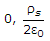B.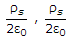C.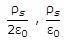D.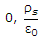Explanation:

In case of capacitor, the charges on two plates of capacitor are of opposite sign.

We know that electric field due to infinite sheet charge with density rs is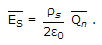Outside the capacitor, electric field intensities due to upper plate and lower plate cancel each other.

Eoutside = 0

Inside the capacitor, the electric field intensities due to upper and lower plate add together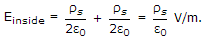#### Current Affairs 2022

Interview Questions and Answers